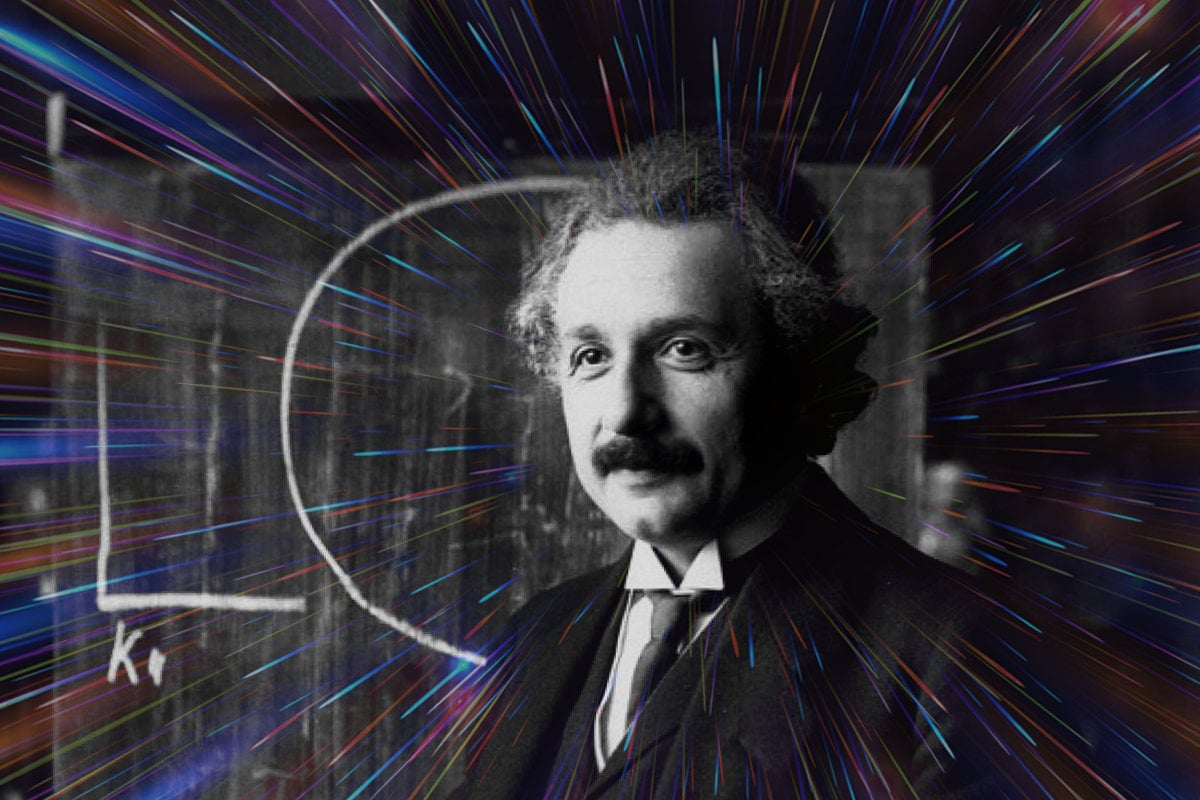# What does Einstein’s E=mc2 mean?

What does Einstein’s famous equation E= mc2 actually mean?

## What equals what what?

Early in the nineteenth century, Albert Einstein, while working as a clerk in a patent office, was inspired to propose a relationship between mass (the m), and energy (the E).

His E=mc2 equation just states, in basic mathematical terms, that there is a simple, constant correlation between mass and energy.

If you were to see an equation that represented the relationship between, say, feet and inches, one would see something like this:

The number of inches = 12 times the number of feet

Mathematically, you would express this relationship as I = 12 F. In this case, the number of inches equals 12 times the number of feet (F). (Mathematicians leave off the multiplication sign — it is understood to be there.)

Another identical equation for the same relationship is feet = inches/12; or that the number of feet equals the number of inches divided by 12.

So if you are looking at 24 inches, you would determine the number of feet, simply dividing by 12. Alternatively, the number of inches can be found by multiplying the number of feet by 12. We all have done this many times in our daily life.

For instance: Let’s say that you need to make sure there’s enough room on your desk for a printer and your computer monitor. So how many inches across is your three-foot wide desk? Multiply 3 times 12, and you get 36.

## E = mc^2: It’s all about a relationship

Einstein’s equation, similarly, represents the constant mathematical relationship between energy (the E in the equation), and mass (the m in the equation).

In our “feet/inches” example, the constant multiplier was 12. In Einstein’s equation, the constant multiplier is also a number, represented as c2 (called “c squared”). It is not a common number, and not one that most people are familiar with. It’s extremely large, and much easier to write it down and work with it if it’s simply represented by two characters.

The number we’re talking about is determined by multiplying the speed of light (which is approximately 186,000 miles per second — meaning it could go around the world 7-1/2 times per second) by itself.

This means that the number is 186,000 times 186,000. (The number actually used in Einstein’s equation was in kilometers per second. That’s approximately 300,000 kilometers per second times 300,000 kilometers per second — which equals 90,000,000,000 [90 billion], or, simply, c2.)

So Einstein’s equation says that to determine the amount of concentrated energy (E) in a unit of mass (m), we multiply by 90 billion.

Same type of equation as “feet/inches” — but the multiplier, instead of 12, is 90 billion. (There are some additional dimensional figures from the “squared” numbers which enter in, but the basic relationship is the 1 to 90 billion.)

## And the point?

So what does this all mean? It means that the amount of concentrated energy in any mass is enormous! This relationship has been verified many times in experimental physics laboratories.

For instance, in an atomic bomb explosion, if you compare the amount of uranium mass that is used to create the “fission” ( the process that creates the enormous energy release in an atom bomb), with the amount of mass left over after the explosion, physicists find that some of the mass (m) has disappeared!

Where did it go? It got converted to energy: E.

When physicists measured the loss of mass (m), they multiplied the loss of mass by Einstein’s “c squared” number — and, lo and behold, got an answer for the amount of energy (E) released… which was exactly what Einstein’s equation predicted.

## The power of thought

The amazing thing about Einstein’s equation is that it was not the result of fitting the results of an experiment to a mathematical formula, but instead was the result of a “thought” experiment — an inspired bit of brain work! Einstein never did an experiment or worked in a scientific laboratory — his breakthroughs were the result of an inspired thought.